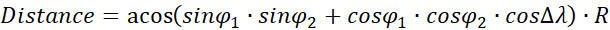You might think "as the crow flies" distance is nothing more than a simple hypotenuse calculation back from geometry class. Unfortunately, not. You should work with either decimal degrees or degree-minute-second (DMS) notation due to the curvy structure of Earth. This means a lot of formulas to deal with.

Also, you need coordinates (latitude/longitude) of each city you want to put in a calculation.

Thankfully, we have workarounds for both problems. In this article, we are going to show how to calculate as-the-crow-flies distance between two cities in Excel with help of LAMBDA function and data types.

## The formula of calculating distance by longitude and latitude

In our example, we are using the great-circle distance method which uses the shortest path, also known as, as the crow flies, between two points corresponding to an arc linking two points on a sphere. The method uses Spherical law of cosines a theorem relating the sides and angles of spherical triangles.where

• R: Radius of the earth

The coordinates are represented in two forms:

• As a string including degree, minute and second
• As a numerical value of decimal degrees

Converting degrees, minutes and seconds to decimal degrees:

Decimal Degrees = Degrees + (Minutes + Seconds / 60) / 60

Radians = Decimal Degrees * PI / 180

Because deg-min-sec notation will make things more confusing, we will use decimal degrees going forward. This is the Excel formula for decimal degrees:

```ACOS(
SIN(Place1_Lat * PI() / 180) *
SIN(Place2_Lat * PI() / 180) +
COS(Place1_Lat * PI() / 180) *
COS(Place2_Lat * PI() / 180) *
COS(Place2_Lon * PI() / 180 - Place1_Lon * PI() / 180)

Obviously, no one wants to deal with this kind of formula more than one time. Instead, you can use the LAMBDA function to define this formula once in a named range and use the named range like a UDF (user-defined function). Let's see what the LAMBDA function is and how you can use it.

LAMBDA Function

The LAMBDA function is a game changer feature that allows you to create your own functions without any VBA, macro, or JavaScript knowledge. Since its unique nature, the function has a unique use case.

• A LAMBDA formula should be written in a named range. That named range's name will be your custom function's name.
• You need to type parameter names before the function itself. Such as, a and b are the parameters and the latter operation is the function itself: =LAMBDA(a, b, a-b)
• LAMBDA functions can be called recursively.

LAMBDA([parameter1, parameter2, …,] calculation)

For detailed information, please visit the following page: Excel LAMBDA Function

The LAMBDA function requires a Microsoft 365 subscription.

## Calculating distance by longitude and latitude with LAMBDA Function

Let's create our custom function as described above. The distance formula needs 4 arguments, if we use a static value for the radius of earth, e.g., 6,371 km.

```=LAMBDA(Place1_Lat, Place1_Lon, Place2_Lat, Place2_Lon,
ACOS(
SIN(Place1_Lat * PI() / 180) * SIN(Place2_Lat * PI() / 180) +
COS(Place1_Lat * PI() / 180) * COS(Place2_Lat * PI() / 180) *
COS(Place2_Lon * PI() / 180 - Place1_Lon * PI() / 180)
) * 6371
)```Once created, you can re-use your custom function anywhere in this workbook. Excel even displays IntelliSense box for this function.Tip: You can use Excel's RADIANS function instead of multiplying by PI() / 180.

## Data Types

Let us introduce you to an Excel feature called "Data Types". The Data Types feature allows pulling data dynamically from online sources. Geographical data like countries and cities are two of them. This feature will relieve you from gathering coordinate data beforehand.

Note: Data Types have been released to all Microsoft 365 subscribers on March 28, 2019. Thus, you need to be a Microsoft 365 subscriber to access this feature.

You can find the feature button under the Data tab of the Ribbon.

All you need to do is to select cells that contain a city name and click the Geography icon. You will see an icon will be added to city names.Once added, you can see options like population, time zone, and more, including latitude and longitude by putting a dot (.) after the cell's reference.Since you can get lat/lon values from a single data-type cell why do you need four arguments in your custom function? You can simplify decrease your argument number to two like below:

```=LAMBDA(City1, City2,
ACOS(
SIN(City1.Latitude * PI() / 180) *
SIN(City2.Latitude * PI() / 180) +
COS(City1.Latitude * PI() / 180) *
COS(City2.Latitude * PI() / 180) *
COS(
City1.Longitude * PI() / 180 -
City2.Longitude * PI() / 180
)
) * 6371
) ```

We named our formula "DistanceByCity":